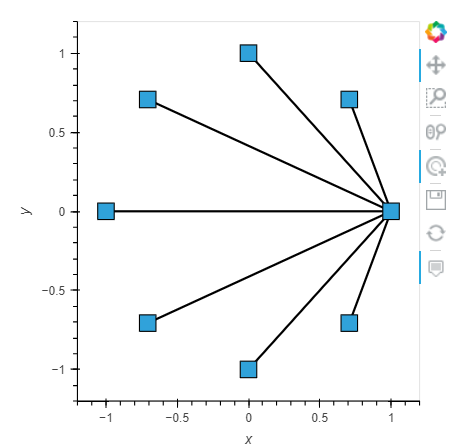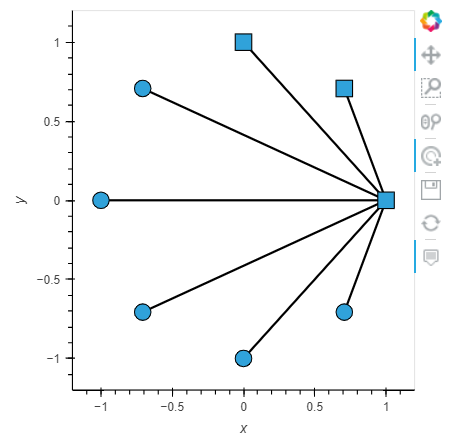# Change the node shape of a network graph

Hello,

I’m searching for a way to change the node shapes of a holoviews network graph (e.g. into triangles or squares instead of the default circles).
Currently, I am using “.opts(node_color= …)” to change the color of the nodes, but I couln’t find a similar parameter for the node shape. Additionally, if possible, I would like to make the outline of the nodes dotted.
If anyone has any tips on how to do this, please let me know. Thanks in advance!

node_marker = ‘square’ works; found it via hv.help(hv.Graph)

``````#adaped from example
import numpy as np
import holoviews as hv
from holoviews import opts
hv.extension('bokeh')
opts.defaults(opts.Graph(width=400, height=400))
# Declare abstract edges
N = 8
node_indices = np.arange(N)
source = np.zeros(N)
target = node_indices

simple_graph = hv.Graph(((source, target),)).opts(node_marker='square')
simple_graph
``````For other markers, check the bokeh scatter docs: Scatter — Bokeh 2.4.1 Documentation

Also… if you want to map the property to data, looks like this works:

``````import numpy as np
import holoviews as hv
from holoviews import opts
import networkx as nx
hv.extension('bokeh')
opts.defaults(opts.Graph(width=400, height=400))

# Declare abstract edges
N = 8
node_indices = np.arange(N)
source = np.zeros(N)
target = node_indices

G = nx.DiGraph()

for t in target: